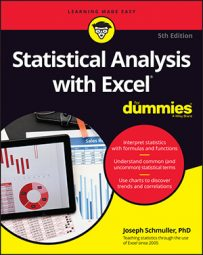##### Statistical Analysis with Excel For DummiesExcel provides functions that help you with factorials, permutations, and combinations. In the world of statistical analysis, these can be very useful. Keep reading to find out how to use these functions.

## FACT

`FACT`, which computes factorials, is surprisingly not categorized as Statistical. Instead, you'll find it on the Math & Trig Functions menu. It's easy to use. Supply it with a number, and it returns the factorial. Here are the steps:
1. Select a cell for `FACT`'s answer.
2. From the Math & Trig Functions menu, select `FACT` to open its Function Arguments dialog box.
3. In the Function Arguments dialog box, enter the appropriate value for the argument. In the Number box, type the number whose factorial you want to compute. The answer appears in the dialog box. If you enter 5, for example, 120 appears.
4. Click OK to put the answer into the selected cell.

## PERMUT and PERMUTIONA

`PERMUT` enables you to calculate NPr. Here's how to use it to find 26P5, the number of 5-letter sequences (no repeating letters) that you can create from the 26 letters of the alphabet. In a permutation, remember, abcde is considered different from bcdae. Follow these steps:
1. Select a cell for `PERMUT`'s answer.
2. From the Statistical Functions menu, select `PERMUT` to open its Function Arguments dialog box.
3. In the Function Arguments dialog box, type the appropriate values for the arguments. In the Number box, enter the N in NPr. For this example, N is 26. In the Number_chosen box, enter the r in NPr. That would be 5. With values entered for both arguments, the answer appears in the dialog box. For this example, the answer is 7893600.
4. Click OK to put the answer into the selected cell.

`PERMUTIONA` does the same thing, but with repetitions allowed. Its Function Arguments dialog box looks exactly like the one for `PERMUT`. Its answer is equivalent to Nr. For this example, by the way, that answer is 1181376.

## COMBIN and COMBINA

`COMBIN` works pretty much the same way as `PERMUT`. Excel categorizes `COMBIN` and `COMBINA` as Math & Trig functions.

Here's how you use them to find 26C5, the number of ways to construct a 5-letter sequence (no repeating letters) from the 26 letters of the alphabet. In a combination, abcde is considered equivalent to bcdae.

1. Select a cell for `COMBIN`
2. From the Math & Trig Functions menu, select `COMBIN` to open its Function Arguments dialog box.
3. In the Function Arguments dialog box, type the appropriate values for the arguments. In the Number box, enter the N in NCr. Once again, N is 26. In the Number_chosen box, enter the r in NCr. And again, r is 5. With values entered for both arguments, the answer appears in the dialog box. For this example, the answer is 65870.
4. Click OK to put the answer into the selected cell.
If you allow repetitions, use `COMBINA`. Its Function Arguments dialog box looks just like `COMBIN`’s. For this example, its answer is equivalent to 30C25 (142506).